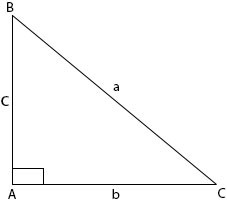### Trigonometry (I)

Mathematics SSS 1 Third Term

WEEK 5

Trigonometry (I)

Performance Objectives

Students should be able to;

1. Identify ratios forming cosine and tangent of marked angle
2. Draw right angle triangles
3. Use right-angle triangles to solve problems involving calculations of lengths

Basic Trigonometric Ratios (Sine, Cosine, Tangent, with respect to right-angled triangles)

Content

These trigonometric ratios are applicable to the right-angled triangle. A right-angle triangle is 900. Thus the remaining two angles add up to 900 since every triangle contains two right angles.In ∆ABC, B + C = 900.

Such angles whose sum is 900 are said to be complementary angles. While capital letters are used for angles, small (lower case) letters are used for sides. Notice that the side opposite A is labelled a, the one opposite B is labelled b etc.

.... View More